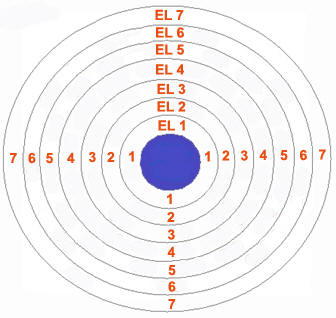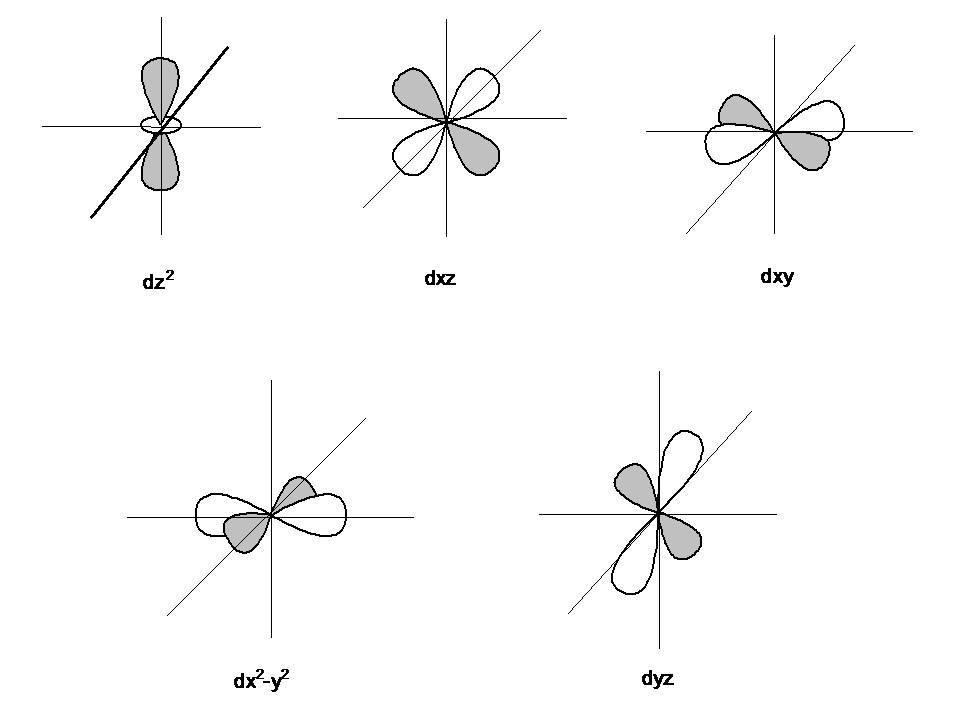# Difference Between Orbit and Orbital in Atomic Theory

Orbit is a term that is mostly discussed in astronomical studies, where it defines the elliptical path on which planets revolve around stars, just like earth and other planets revolve around the sun. However, orbit in chemistry is a bit different from an astronomical orbit, but still denotes a path. In chemistry, orbit is a definite circular path, in which electrons of an atom revolve around the nucleus. The necessary centripetal force required to keep the negatively charged electron in the orbit is provided by the positively charged nucleus, present at the centre of the atom. In atomic theory, both “orbit” and “orbital” are used to define the path of electrons and often confuse people, but they are two different terms that can easily be differentiated.

An orbit is a two-dimensional circular path, where as an orbital represents a three-dimensional path around the nucleus. The former, being a two-dimensional space, represents the planer movement of electrons around the nucleus, while the latter is used to represent three-dimensional movement.

In an orbit, the exact location of an electron in an atom can be defined, whereas an orbital does not indicate the exact position of electrons. According to Heisenberg’s Uncertainty Principle, “One can never know with perfect accuracy both of those two important factors which determine the movement of one of the smallest particles (electrons)—its position and its velocity. It is impossible to determine accurately both the position and the direction and speed of a particle or electron at the same instant.”

An orbit can accommodate 2n2 electrons in it, where n is the number of the orbit. Orbitals on the other hand can accommodate 2, 6, 10 and 16 electrons.

### Instructions

• 1

Orbit

As postulated by Bohr, “an orbit is a definite circular path at a definite distance from the nucleus in which the electrons revolve round the nucleus”. Orbits are represented by the capital letters K, L, M, N…. etc. K is the first shell around the nucleus and can accommodate only two electrons, L can accommodate 8 electrons and so on. The electrons in a shell follow the octet rule, which means all the orbits except the K shell, should contain at least eight electrons for an atom to remain in neutral state.

Image courtesy: saburchill.com• 2

Orbital

As postulated by the wave nature of an electron, “an orbital is a three-dimensional region around the nucleus within which the probability of finding an electron is maximum”. Orbitals are actually the sub shells around the nucleus and are categorized as s, p, d and f orbitals.

- s orbital – 2 electrons
- p orbital – 6 electrons
- d orbital – 10 electrons
- f orbital – 16 electrons

Image courtesy: chemwiki.ucdavis.edu•